• 上一篇博客画了一张核密度函数，但是搜了半天也没找到怎么分析这个。求人不如求自己，这篇来分析一下这个核密度函数和核密度。说的不对的地方还请大神指出。 概率密度 从数学上看，分布函数F(x)=P(X<x)，...
前言
上一篇博客画了一张核密度函数，但是搜了半天也没找到怎么分析这个图。求人不如求自己，这篇来分析一下这个核密度函数和核密度图。说的不对的地方还请大神指出。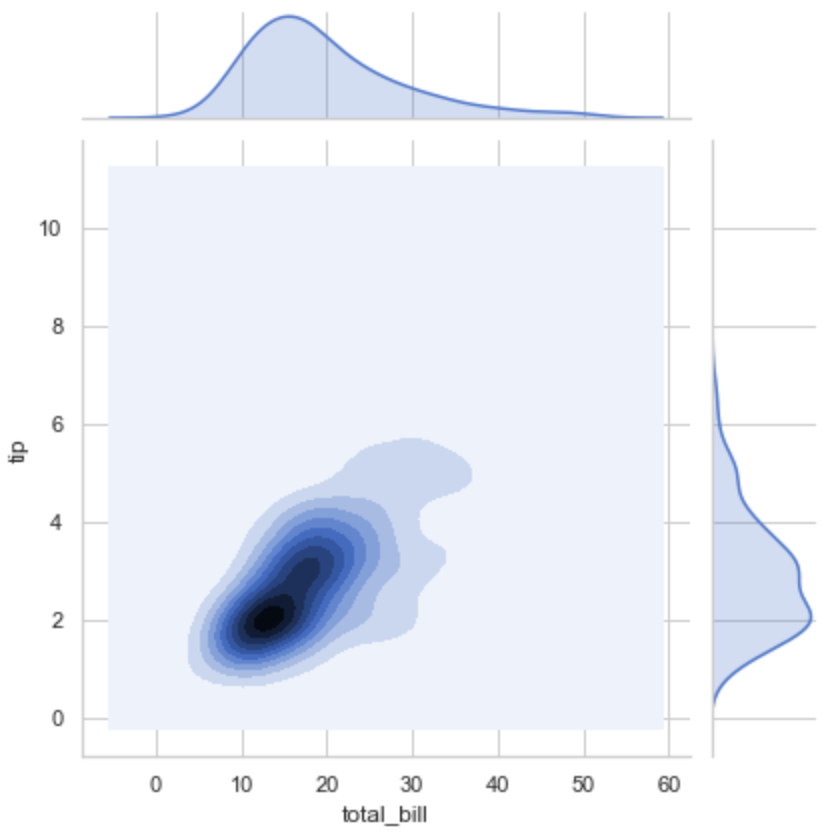概率密度
从数学上看，分布函数F(x)=P(X<x)，表示随机变量X的值小百于x的概率。这个意义很容易理解。
概率密度f(x)是F(x)在x处的关于x的一阶导数，即变化率。如果在某一x附近取非常小的一个邻域Δx，那么，随机度变量X落在(x, x+Δx)内的概率约为f(x)Δx，即P(x<X<x+Δx)≈f(x)Δx。
换句话说，概率密度f(x)是X落在x处“单位宽度”内的概率。“密度”一词可以由此理解。
For me:
概率密度也可以理解为概率密度函数道是概率分布函数的一阶导函数，斜率
已知连续型随机变量的密度函数，可以通过讨论及定积分的计算求出其分布函数；当已知连续型随机变量的分布函数时，对其求导就可得到密度函数。
对离散型随机变量而言，如果知道权其概率分布（分布列），也可求出其分布函数；当然，当知道其分布函数时也可求出概率分布。
核密度估计
核密度估计的原理其实是很简单的。在我们对某一事物的概率分布的情况下。如果某一个数在观察中出现了，我们可以认为这个数的概率密度很比大，和这个数比较近的数的概率密度也会比较大，而那些离这个数远的数的概率密度会比较小。基于这种想法，针对观察中的第一个数，我们都可以f(x-xi)去拟合我们想象中的那个远小近大概率密度。当然其实也可以用其他对称的函数。针对每一个观察中出现的数拟合出多个概率密度分布函数之后，取平均。如果某些数是比较重要，某些数反之，则可以取加权平均。
总结
由原理可见，分析核密度函数时主要是要观察其面积，而不是取值，因为对应的取值是一个概率密度，只有与变量相乘才能得到该变量的概率取值。


展开全文python 机器学习 数据分析 算法
• 在本篇博客中，我将与众位看官分享我自己写的关于用核密度函数加权的直方的计算 欢饮批评指正！！！ 核密度函数加权直方在基于Mean shift的跟踪算法中经常用到。请客官查看我的博客： 样本均值漂移的原理Mean...
在本篇博客中，我将与众位看官分享我自己写的关于用核密度函数加权的直方图的计算

欢饮批评指正！！！

核密度函数加权直方图在基于Mean shift的跟踪算法中经常用到。请客官查看我的博客：

样本均值漂移的原理 Mean Shift 原理

常用的核密度函数有

EpanechnikovKernal：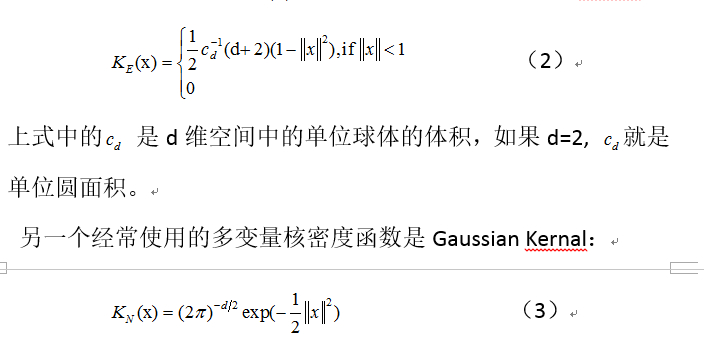在Mean Shift的迭代过程中用的是上述两个函数的截面函数：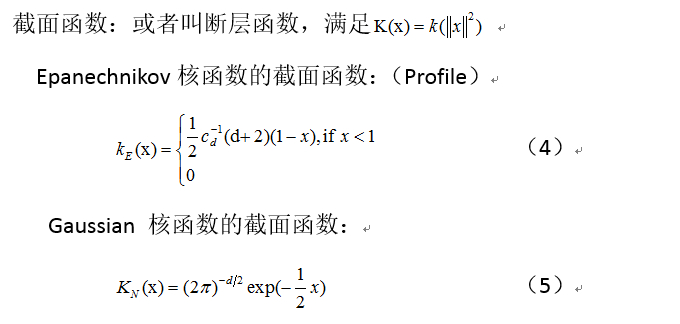下面是这两个函数(4)和(5)的程序实现：

/*核函数：Epanechnikov Kernal

center: 核函数中心坐标

xy : 在核区域内的某一个点

hx,hy : 核区域在x方向和y方向的半径（或叫 带宽）

*/

static float EpanechnikovKernalFunc(Point2f& center,Point2f& xy,int hx,int hy)

{

//计算点xy到中心center的归一化距离

float distx = (xy.x - center.x)/hx;

float disty = (xy.y - center.y)/hy;

float dist_square = distx*distx + disty*disty;//距离平方

float result = 0.f; //函数要返回的结果

//核区域就是以hx和hy为边长的矩形的内接圆，在该内接圆中的点

//的函数值都不为0，若距离超过核区域，则返回0，

//距离center越近，函数值越大

if(dist_square>=1) 

{

result = 0.f; 

}

else

{

//float Cd = CV_PI; //单位圆面积 pi*R^2 ,R==1

float Cd_inv = 0.318309886f; // == 1.0/Cd;单位圆的面积的倒数

int dimension = 2; //坐标的维数

//Epanechnikov核函数的截面断层函数：profile

result = 0.5f*Cd_inv*( dimension + 2 )*( 1 - dist_square );

}

return result;

}

/*核函数：Gaussian Kernal

center: 核函数中心坐标

xy : 在核区域内的某一个点

hx,hy : 核区域在x方向和y方向的半径（或叫 带宽）

*/

static float GaussianKernalFunc(Point2f& center,Point2f& xy,int hx,int hy)

{

//计算点xy到中心center的归一化距离

float distx = (xy.x - center.x)/hx;

float disty = (xy.y - center.y)/hy;

float dist_square = distx*distx + disty*disty;//距离平方

float result = 0.f; //函数要返回的结果

//核区域就是以hx和hy为边长的矩形的内接圆，在该内接圆中的点

//的函数值都不为0，若距离超过核区域，则返回0，

//距离center越近，函数值越大

if(dist_square>=1) 

{

result = 0.f; 

}

else

{

float Cd = 6.2831853072f; // ==2*CV_PI

float Cd_inv = 1.0f/Cd;

int dimension = 2; //坐标的维数

result = Cd_inv*expf( -0.5f*dist_square ); //高斯核函数的截面断层函数：profile

}

return result;

}

下面是调用上述核函数实现的一维加权直方图统计函数

该函数中的最后一个参数是函数指针类型的参数，用于指定到底用哪个核函数

KernalFunc的定义如下：

//定义一个核函数指针，该函数指针可以指向任意一个符合参数标准的核函数
typedef float (*KernalFunc)(Point2f& center,Point2f& xy,int hx,int hy);

//计算图像一维加权直方图的函数

static void myCalcWeightedHist1D( const Mat& image, vector

& channels,OutputArray _hist, 

int dims, const int* histSize,const float** ranges,KernalFunc kernal)

{

//KernalFunc kernal;//声明一个KernalFunc类型的函数指针变量 

int calc_channels = channels.size();//image图像中指定要被统计计算的通道的个数

int img_channel = image.channels(); //image图像的总的通道个数

if(img_channel < channels[calc_channels - 1] + 1 )

{

printf("channels中的最大通道索引数不能超过images的通道数\n");

getchar();

}

if(image.depth() != CV_8U)

{

printf("该函数仅支持CV_8U类的图像\n");

getchar();

}

if(dims != calc_channels)

{

printf("被索引的通道数目与直方图的维数不相等\n");

getchar();

}

int img_width = image.cols; //图像宽度

int img_height = image.rows;//图像高度

//图像区域的中心，即核函数中心

Point2f center(0.5f*img_width,0.5f*img_height);

int hx = img_width/2 , hy = img_height/2;//核函数带宽

float NormalConst =0.f; //用于归一化加权系数

//参数_hist是输出参数，保存着计算好的直方图，一维直方图是histSize行1列的矩阵

_hist.create(dims, histSize, CV_32F); //为输出直方图开辟空间

Mat hist = _hist.getMat(); 

hist.flags = (hist.flags & ~CV_MAT_TYPE_MASK)|CV_32S;//直方图矩阵的类型信息 

hist = Scalar(0.f); //清空原来的数据，当前直方图不累加到原来的直方图上去

int ch = channels[dims-1];//要统计的通道编号 ==: 0 or 1 or 2 

float low_range = ranges ,high_range = ranges;//要计算的直方图区间的上下限

float a = histSize/(high_range - low_range);/* 单位区间内直方图bin的数目*/

float b = -a*low_range;

//外循环按行从上往下扫描 

for(int y=0; y < img_height ; y++ )

{

//指向图像第y行的指针

const uchar* ptr_y = image.ptr

(y);

//内循环从左往右扫描

for(int x=0; x < img_width ; x++)

{

//取出输入图像的第y行第x列第ch通道的像素值

uchar val = ptr_y[x*img_channel + ch];

//灰度值val在输入参数规定的灰度区间内的bin序号的索引值

int idx = cvFloor(val*a + b);//该索引值是数学上线性运算后得到的

float* hist_ptr = hist.ptr

(idx); //指向输出直方图的第idx个bin

float weighted_value = kernal(center,Point2f(x,y),hx,hy); //（x,y）位置处的加权值

(*hist_ptr) += weighted_value; //加权累计直方图的每一个bin

NormalConst += weighted_value; //加权系数归一化因子

}

}

float NomalConst_inv = 1.0f/(NormalConst + DBL_EPSILON);

//归一化加权直方图

for(int ii=0; ii < histSize; ii++)

{

float* hist_ptr = hist.ptr

(ii); //指向输出直方图的第ii个bin

*hist_ptr *= NomalConst_inv;

}

return;

}

下面是测试上述各个小函数的主函数：

说明：在主函数中，计算了三种不同的一维直方图。

一种是非加权直方图，它的计算函数可以参见我的博客：自己编写的直方图函数

第二种是Epanikov 核函数加权的直方图

第三种是Gaussian核函数加权的直方图

主函数：

//基于核函数的加权直方图计算

#include "stdafx.h"

#include 

#include 

#include 

using namespace std;

using namespace cv;

Mat image; //母图像

Mat roi_hist; //兴趣区域图像块的直方图

Mat roi_whist1,roi_whist2;//兴趣区域图像块的加权直方图

Mat roi_img; //兴趣区域的图像块

Rect roi_rect(162,159,100,122); //ROI图像块在原图的真正区域

Mat ms_hist; //由MeanShift给出的目标区域的直方图

Mat ProbImg; //反射概率分布图像

bool selectObject = false; //selectObject的初始值为false,

int calculateHist = 0; //calculateHist的初值是0

bool showHist = false;

Point origin;

Rect selection;

//指定要统计的通道编号

vector

selected_channel(1,0);

//设定直方图中bin的数目

int histSize = 128;

//设定取值范围

float range[] = {0,256};//灰度特征的范围都是[0,256)

const float* histRange = {range}; 

bool uniform = true; //均匀分布，

bool accumu = false;//无累加

int hist_w = 400; int hist_h = 400;//直方图图像的宽度和高度

int bin_w = cvRound( (double) hist_w/histSize );//直方图中一个矩形条纹的宽度

// 创建直方图画布 

Mat histImage( hist_w, hist_h, CV_8UC3, Scalar( 0,0,0) );//创建画布图像

//用鼠标选择目标图像区域

static void onMouse( int event, int x, int y, int, void* );

//该函数用于计算给定矩形图像块的加权直方图

static void CalculateWeightedHistogram(const Mat& img,vector

& channels, Mat& _hist,int weighted_method); //该函数用于计算给定矩形图像块的非加权直方图 static void CalculateHistogram(const Mat& img,vector

& channels, Mat& _hist); //自己写的计算图像一维直方图的函数 void myCalcHist1D( const Mat& image, vector

& channels,OutputArray _hist, int dims, const int* histSize,const float** ranges); //定义一个核函数指针，该函数指针可以指向任意一个标准的核函数 typedef float (*KernalFunc)(Point2f& center,Point2f& xy,int hx,int hy); //计算图像一维加权直方图的函数 static void myCalcWeightedHist1D( const Mat& image, vector

& channels,OutputArray _hist, int dims, const int* histSize,const float** ranges,KernalFunc kernal); /*核函数：Epanechnikov Kernal*/ static float EpanechnikovKernalFunc(Point2f& center,Point2f& xy,int hx,int hy); /*核函数：Gaussian Kernal */ static float GaussianKernalFunc(Point2f& center,Point2f& xy,int hx,int hy); //绘制直方图 void DrawHistogramImage( Mat& _hist , Scalar& color); int _tmain(int argc, _TCHAR* argv[]) { string filename = "meinv1.jpg"; string winname = "MainWindow"; namedWindow(winname,1); namedWindow("Hist Image",1); namedWindow("ROI Image",1); //为 Histogram Demo窗口添加鼠标响应函数，用于选择图像块 setMouseCallback( "MainWindow", onMouse, 0 ); Mat src, dst;//声明原始图像和目标图像 /// 装载图像 src = imread( filename, 1 ); if( !src.data ) //判断图像是否成功读取 { return -1; } //src.copyTo(image); cvtColor(src,image,CV_BGR2GRAY); for(;;) { ///循环显示读入的图像 imshow(winname,src); if(calculateHist) { roi_img = image(selection);//提取鼠标选中的图像块 imshow("ROI Image",roi_img);//显示roi 图像块 calculateHist = 0; //计算选中的图像块的直方图 CalculateHistogram(roi_img,selected_channel,roi_hist); //计算选中图像块的加权直方图 int weighted_method = 0; //指定加权核函数的种类 CalculateWeightedHistogram(roi_img,selected_channel,roi_whist1,weighted_method); weighted_method = 1; //使用高斯核函数 CalculateWeightedHistogram(roi_img,selected_channel,roi_whist2,weighted_method); //清空画布 histImage = Scalar::all(0); //绘制直方图 DrawHistogramImage(roi_hist,Scalar(0,0,255)); //绘制加权直方图 DrawHistogramImage(roi_whist1,Scalar(0,255,0)); //绘制加权直方图 DrawHistogramImage(roi_whist2,Scalar(255,0,0)); cout<<"红色曲线： 未加权直方图"<

0 && selection.height > 0 ) calculateHist = 1; //当在第一帧用鼠标选定了合适的目标跟踪窗口后，calculateHist的值置为 1 //selection = roi_rect; //放开这一句注释，则鼠标选取被无效化，使用roi_rect的默认位置作为roi区域 cout<<"选中的矩形区域为： "<

<

& channels, Mat& _hist,int weighted_method) { //调用自己写的函数计算选中图像块的加权直方图 KernalFunc kernal;//声明一个KernalFunc类型的函数指针变量 //根据加权参数指定具体的核函数 if(weighted_method == 0) kernal = EpanechnikovKernalFunc; //调用Epanechnikov核函数 else if(weighted_method == 1) kernal = GaussianKernalFunc; //调用高斯Gaussian核函数 myCalcWeightedHist1D(img,channels,_hist,1,&histSize,&histRange,kernal); /// 将直方图归一化到范围 [ 0, 1] normalize(_hist, _hist, 0, 1, NORM_MINMAX, -1, Mat() ); } //该函数用于计算给定矩形图像块的非加权直方图 static void CalculateHistogram(const Mat& img,vector

& channels, Mat& _hist) { //调用OpenCV函数计算选中图像块的灰度直方图 //calcHist(&img,1,0,Mat(),_hist,1,&histSize,&histRange,uniform,accumu); //调用自己写的函数计算选中图像块的灰度直方图 myCalcHist1D(img,channels,_hist,1,&histSize,&histRange); /// 将直方图归一化到范围 [ 0, 1] normalize(_hist, _hist, 0, 1, NORM_MINMAX, -1, Mat() ); } //绘制直方图 void DrawHistogramImage( Mat& _hist , Scalar& color) { //用于绘图的直方图矩阵数组 Mat draw_hist; /// 将直方图归一化到范围 [ 0, histImage.rows ] normalize( _hist , draw_hist , 0, histImage.rows, NORM_MINMAX, -1, Mat() ); /// 在直方图画布上画出直方图 /*histImage = Scalar::all(0);*/ for(int i=1;i<=histSize;i++) { //矩形图表示 /* rectangle( histImage,Point((i-1)*bin_w,hist_h),Point(i*bin_w,hist_h-cvRound(draw_hist.at

(i-1))),Scalar(0,0,255),1,8,0); */ //折线图表示 line( histImage, Point( bin_w*(i-1), hist_h - cvRound(draw_hist.at

(i-1)) ) , Point( bin_w*(i), hist_h - cvRound(draw_hist.at

(i)) ), color, 1, 8, 0 ); } /// 显示直方图 imshow("Hist Image", histImage ); } /** 计算给定图像的一维直方图，图像类型必须是CV_8UCx的,x = 1,or 2, or 3 or ...., channels规定了通道索引顺序，对于1D直方图,channels数组只能有一个值，且必须 < x */ void myCalcHist1D( const Mat& image, vector

& channels,OutputArray _hist, int dims, const int* histSize,const float** ranges) { int calc_channels = channels.size();//image图像中指定要被统计计算的通道的个数 int img_channel = image.channels(); //image图像的总的通道个数 if(img_channel < channels[calc_channels - 1] + 1 ) { printf("channels中的最大通道索引数不能超过images的通道数\n"); getchar(); } if(image.depth() != CV_8U) { printf("该函数仅支持CV_8U类的图像\n"); getchar(); } if(dims != calc_channels) { printf("被索引的通道数目与直方图的维数不相等\n"); getchar(); } int img_width = image.cols; //图像宽度 int img_height = image.rows;//图像高度 //参数_hist是输出参数，保存着计算好的直方图，一维直方图是histSize行1列的矩阵 _hist.create(dims, histSize, CV_32F); //为输出直方图开辟空间 Mat hist = _hist.getMat(); hist.flags = (hist.flags & ~CV_MAT_TYPE_MASK)|CV_32S;//直方图矩阵的类型信息 hist = Scalar(0.f); //清空原来的数据，当前直方图不累加到原来的直方图上去 int ch = channels[dims-1];//要统计的通道编号 ==: 0 or 1 or 2 float low_range = ranges ,high_range = ranges;//要计算的直方图区间的上下限 float a = histSize/(high_range - low_range);/* 单位区间内直方图bin的数目*/ float b = -a*low_range; //外循环按行从上往下扫描 for(int y=0; y < img_height ; y++ ) { //指向图像第y行的指针 const uchar* ptr_y = image.ptr

(y); //内循环从左往右扫描 for(int x=0; x < img_width ; x++) { //取出输入图像的第y行第x列第ch通道的像素值 uchar val = ptr_y[x*img_channel + ch]; //灰度值val在输入参数规定的灰度区间内的bin序号的索引值 int idx = cvFloor(val*a + b);//该索引值是数学上线性运算后得到的 float* hist_ptr = hist.ptr

(idx); //指向输出直方图的第idx个bin (*hist_ptr)++; //累计idx对应的直方图bin上的数量 } } return; } /*核函数：Epanechnikov Kernal center: 核函数中心坐标 xy : 在核区域内的某一个点 hx,hy : 核区域在x方向和y方向的半径（或叫 带宽） */ static float EpanechnikovKernalFunc(Point2f& center,Point2f& xy,int hx,int hy) { //计算点xy到中心center的归一化距离 float distx = (xy.x - center.x)/hx; float disty = (xy.y - center.y)/hy; float dist_square = distx*distx + disty*disty;//距离平方 float result = 0.f; //函数要返回的结果 //核区域就是以hx和hy为边长的矩形的内接圆，在该内接圆中的点 //的函数值都不为0，若距离超过核区域，则返回0， //距离center越近，函数值越大 if(dist_square>=1) { result = 0.f; } else { //float Cd = CV_PI; //单位圆面积 pi*R^2 ,R==1 float Cd_inv = 0.318309886f; // == 1.0/Cd;单位圆的面积的倒数 int dimension = 2; //坐标的维数 //Epanechnikov核函数的截面断层函数：profile result = 0.5f*Cd_inv*( dimension + 2 )*( 1 - dist_square ); } return result; } /*核函数：Gaussian Kernal center: 核函数中心坐标 xy : 在核区域内的某一个点 hx,hy : 核区域在x方向和y方向的半径（或叫 带宽） */ static float GaussianKernalFunc(Point2f& center,Point2f& xy,int hx,int hy) { //计算点xy到中心center的归一化距离 float distx = (xy.x - center.x)/hx; float disty = (xy.y - center.y)/hy; float dist_square = distx*distx + disty*disty;//距离平方 float result = 0.f; //函数要返回的结果 //核区域就是以hx和hy为边长的矩形的内接圆，在该内接圆中的点 //的函数值都不为0，若距离超过核区域，则返回0， //距离center越近，函数值越大 if(dist_square>=1) { result = 0.f; } else { float Cd = 6.2831853072f; // ==2*CV_PI float Cd_inv = 1.0f/Cd; int dimension = 2; //坐标的维数 result = Cd_inv*expf( -0.5f*dist_square ); //高斯核函数的截面断层函数：profile } return result; } //计算图像一维加权直方图的函数 static void myCalcWeightedHist1D( const Mat& image, vector

& channels,OutputArray _hist, int dims, const int* histSize,const float** ranges,KernalFunc kernal) { //KernalFunc kernal;//声明一个KernalFunc类型的函数指针变量 int calc_channels = channels.size();//image图像中指定要被统计计算的通道的个数 int img_channel = image.channels(); //image图像的总的通道个数 if(img_channel < channels[calc_channels - 1] + 1 ) { printf("channels中的最大通道索引数不能超过images的通道数\n"); getchar(); } if(image.depth() != CV_8U) { printf("该函数仅支持CV_8U类的图像\n"); getchar(); } if(dims != calc_channels) { printf("被索引的通道数目与直方图的维数不相等\n"); getchar(); } int img_width = image.cols; //图像宽度 int img_height = image.rows;//图像高度 //图像区域的中心，即核函数中心 Point2f center(0.5f*img_width,0.5f*img_height); int hx = img_width/2 , hy = img_height/2;//核函数带宽 float NormalConst =0.f; //用于归一化加权系数 //参数_hist是输出参数，保存着计算好的直方图，一维直方图是histSize行1列的矩阵 _hist.create(dims, histSize, CV_32F); //为输出直方图开辟空间 Mat hist = _hist.getMat(); hist.flags = (hist.flags & ~CV_MAT_TYPE_MASK)|CV_32S;//直方图矩阵的类型信息 hist = Scalar(0.f); //清空原来的数据，当前直方图不累加到原来的直方图上去 int ch = channels[dims-1];//要统计的通道编号 ==: 0 or 1 or 2 float low_range = ranges ,high_range = ranges;//要计算的直方图区间的上下限 float a = histSize/(high_range - low_range);/* 单位区间内直方图bin的数目*/ float b = -a*low_range; //外循环按行从上往下扫描 for(int y=0; y < img_height ; y++ ) { //指向图像第y行的指针 const uchar* ptr_y = image.ptr

(y); //内循环从左往右扫描 for(int x=0; x < img_width ; x++) { //取出输入图像的第y行第x列第ch通道的像素值 uchar val = ptr_y[x*img_channel + ch]; //灰度值val在输入参数规定的灰度区间内的bin序号的索引值 int idx = cvFloor(val*a + b);//该索引值是数学上线性运算后得到的 float* hist_ptr = hist.ptr

(idx); //指向输出直方图的第idx个bin float weighted_value = kernal(center,Point2f(x,y),hx,hy); //（x,y）位置处的加权值 (*hist_ptr) += weighted_value; //加权累计直方图的每一个bin NormalConst += weighted_value; //加权系数归一化因子 } } float NomalConst_inv = 1.0f/(NormalConst + DBL_EPSILON); //归一化加权直方图 for(int ii=0; ii < histSize; ii++) { float* hist_ptr = hist.ptr

(ii); //指向输出直方图的第ii个bin *hist_ptr *= NomalConst_inv; } return; } 

实验结果：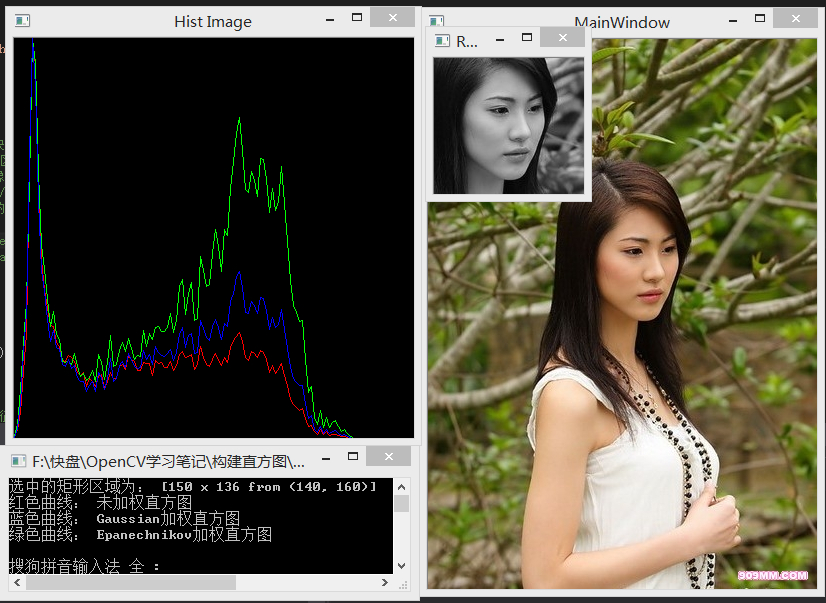上图显示了对美女脸部图像的加权直方图的统计结果(实际计算的时候是在灰度图像上计算的），

红色曲线是未加权直方图，它的中间部分有一个小高突起，是因为美女的脸部灰度值在100到150区间内，

蓝色曲线是高斯加权直方图的结果，可以看到中间的小峰比红色曲线高，这是由于用高斯函数进行了加权，把脸部中间的直方图bin的灰度频率值提高了

绿色曲线是Epanechikov核函数的加权结果，可以看到中间的小峰比蓝色曲线还要高，这是因为Epanechikov核函数比高斯核函数在中心区域更加陡峭所致，所以加权效果也比高斯函数要大的多


展开全文• 直方和和密度函数放在一张上，有两种方式，1.使用pandas自带的工具绘制 2. 使用seaborn库绘制 1.使用pandas自带的工具绘制 要想放在一张上 需要先把直方标准化 #导包 import matplotlib.pyplot as plt ...
将直方图和核密度函数放在一张图上，有两种方式，1.使用pandas自带的工具绘制  2. 使用seaborn库绘制

1.使用pandas自带的工具绘制(设置density = True)

#导包
import matplotlib.pyplot as plt
import numpy as np
import pandas as pd


# 数据加载
data = pd.read_csv('D:/Download/bankpep.csv')
data.head()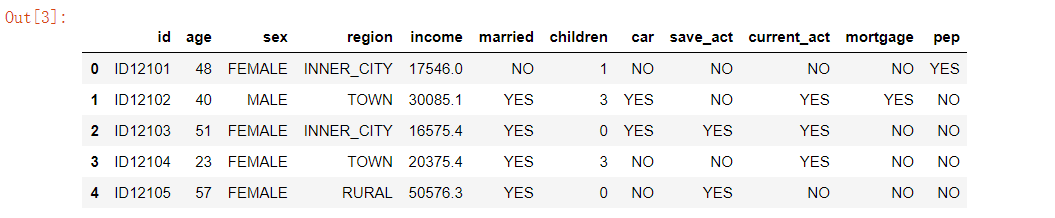# 客户年龄分布的直方图和密度图
data.age.plot.hist(density = True,bins = 9)
data.age.plot(kind='kde',title = "KDE of the distribution of customers' age")
plt.title( "Histogram of the distribution of customers' age")
plt.show()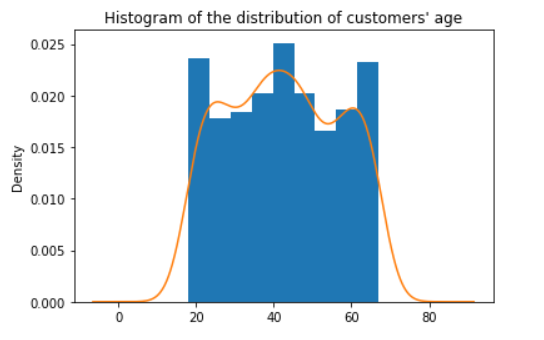2. 使用seaborn库绘制

import seaborn as sns
sns.distplot(data.age)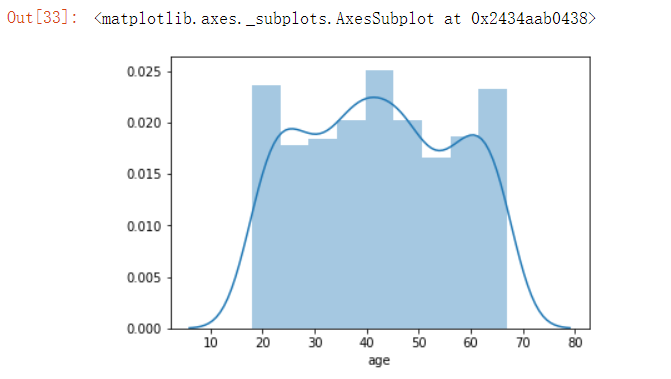欢迎 ： 转发+评论+点赞哟~~

谢谢支持 (*^▽^*)~~！

展开全文python 可视化
• 函数密度估计​kdeplot(),该主要用来拟合并绘制单变量或双变量核密度估计值。 函数原型 seaborn.kdeplot(data, data2=None, shade=False, vertical=False, kernel='gau', bw='scott', ...
核函数密度估计图核函数密度估计图函数原型参数解读案例教程案例地址
核函数密度估计图
核函数密度估计图​kdeplot(),该图主要用来拟合并绘制单变量或双变量核密度估计值。
函数原型
seaborn.kdeplot(data, data2=None, shade=False,
vertical=False, kernel='gau', bw='scott',
gridsize=100, cut=3, clip=None,
legend=True, cumulative=False,
shade_lowest=True, cbar=False,
cbar_ax=None, cbar_kws=None, ax=None, **kwargs)

参数解读
shade:阴影：bool类型
作用：设置曲线下方是否添加阴影，如果为True则在曲线下方添加阴影
(如果数据为双变量则使用填充的轮廓绘制)，若为False则，不绘制阴影

cbar:bool类型
作用：如果为True则绘制双变量KDE图，并添加颜色条

案例教程
案例代码已上传：Github地址
import matplotlib.pyplot as plt
import numpy as np;
import seaborn as sns;
sns.set(color_codes=True)
np.random.seed(10)
#构建数据
mean, cov = [0, 2], [(1, .5), (.5, 1)]
x, y = np.random.multivariate_normal(mean, cov, size=50).T
"""
案例1：
绘制基本的单变量密度曲线图
"""
sns.kdeplot(x)
plt.show()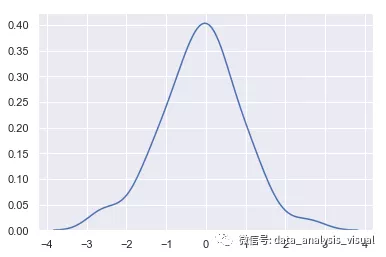import matplotlib.pyplot as plt
import numpy as np;
import seaborn as sns;
sns.set(color_codes=True)
np.random.seed(10)
#构建数据
mean, cov = [0, 2], [(1, .5), (.5, 1)]
x, y = np.random.multivariate_normal(mean, cov, size=50).T
"""
案例2：
绘制密度曲线，并在曲线下着色
通过设置color来设置不同的显示颜色
"""
sns.kdeplot(x, shade=True, color="g")
plt.show()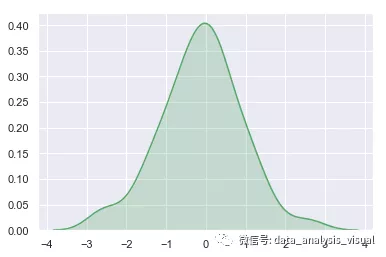import matplotlib.pyplot as plt
import numpy as np;
import seaborn as sns;
sns.set(color_codes=True)
np.random.seed(10)
#构建数据
mean, cov = [0, 2], [(1, .5), (.5, 1)]
x, y = np.random.multivariate_normal(mean, cov, size=50).T
"""
案例3：
绘制双变量密度曲线
"""
sns.kdeplot(x, y)
plt.show()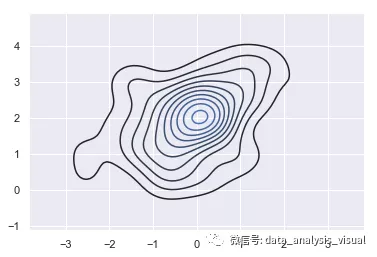import matplotlib.pyplot as plt
import numpy as np;
import seaborn as sns;
sns.set(color_codes=True)
np.random.seed(10)
#构建数据
mean, cov = [0, 2], [(1, .5), (.5, 1)]
x, y = np.random.multivariate_normal(mean, cov, size=50).T
"""
案例 4:
使用轮廓填充
"""
sns.kdeplot(x, y, shade=True,color='r')
plt.show()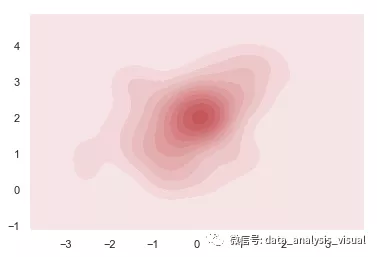import matplotlib.pyplot as plt
import numpy as np;
import seaborn as sns;
sns.set(color_codes=True)
np.random.seed(10)
#构建数据
mean, cov = [0, 2], [(1, .5), (.5, 1)]
x, y = np.random.multivariate_normal(mean, cov, size=50).T
"""
案例5：
使用更多轮廓级别和不同的调色板：
"""
sns.kdeplot(x, y, n_levels=30, cmap="Purples_d")
plt.show()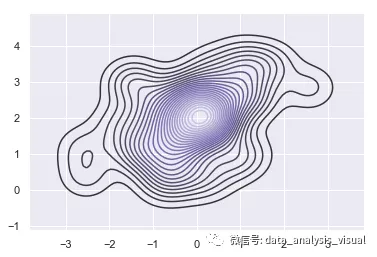import matplotlib.pyplot as plt
import numpy as np;
import seaborn as sns;
sns.set(color_codes=True)
np.random.seed(10)
#构建数据
mean, cov = [0, 2], [(1, .5), (.5, 1)]
x, y = np.random.multivariate_normal(mean, cov, size=50).T
"""
案例6：
给轮廓添加颜色条
"""
sns.kdeplot(x, y, cbar=True)
plt.show()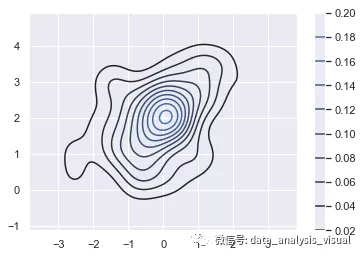import matplotlib.pyplot as plt
import numpy as np;
import seaborn as sns;
# 获取数据
iris = sns.load_dataset("iris")
setosa = iris.loc[iris.species == "setosa"]
virginica = iris.loc[iris.species == "virginica"]
"""
案例7：
绘制多个阴影双变量密度图(这里绘制两个)
"""
sns.kdeplot(setosa.sepal_width, setosa.sepal_length,cmap="Reds", shade=True, shade_lowest=False)

sns.kdeplot(virginica.sepal_width, virginica.sepal_length,cmap="Blues", shade=True, shade_lowest=False)
plt.show()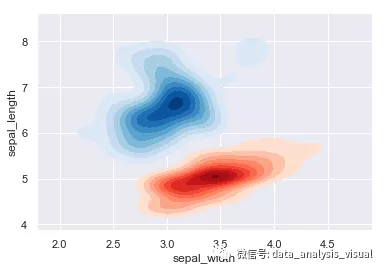案例地址
上述案例代码已上传：Github地址
Github地址https://github.com/Vambooo/SeabornCN
更多技术干货在公众号：数据分析与可视化学研社展开全文数据可视化 python可视化 seaborn可视化
• 核密度函数 与柱状类似，可以使用核密度函数: df.plot.kde(subplots=True,figsize=(5,9)) 它给出了与柱状相似的结果。必须在这里指定一个图形大小，否则图形会在垂直方向上被压得太厉害。 此外，还将subplots...数据可视化
• 核密度图 ...核密度估计（kernel density estimation）是在概率论中用来估计未知的密度函数，属于非参数检验方法之一，由Rosenblatt (1955)和Emanuel Parzen (1962)提出，又名Parzen窗（Parzen windo
• 1. 核密度估计，通过核函数来进行密度估计 2. 六边形分箱（Hexagonal Binning），直接计算不同bin中的点的数量 首先来说一下核密度估计 具体什么是核密度估计建议大家去B站搜索一下，讲的很清楚，大概就是...python 数据可视化
• 参考前文：R绘图笔记 | R语言绘图系统与常见绘图函数及参数前面，介绍过散点绘制，也介绍了柱状的绘制。本文介绍直方核密度估计的绘制。1.直方直方是数值数据分布的精确图形表示...数据可视化 可视化 go ggplot2 数据分析
• ## R之核密度图

万次阅读 2017-12-23 20:22:50
# 用术语来说，核密度估计是用于估计随机变 # 量概率密度函数的一种非参数方法。虽然其数学细节已经超出了本书的范畴，但从总体上讲，核 # 密度不失为一种用来观察连续型变量分布的有效方法。绘制密度的方法（不...
• 核密度图可以看作是概率...法一：seaborn的kdeplot函数专门用于画核密度估计. 参考：https://www.jianshu.com/p/844f66d00ac1 https://yq.aliyun.com/articles/682843 import pandas as pd import numpy ...
• sm包中sm.density.compare函数用于绘制核密度图核密度图是用一条密度曲线而不是通过柱状来展示连续型变量的分布。相比直方，密度的一个优势是可以堆放，可用于比较组间差异。sm.density.compare函数可以直接...
• 在seaborn中使用kdeplot函数绘制核密度图，该章节主要内容有： 基础核密度图绘制 Basic density plot 核密度图的区间控制 Control bandwidth of density plot 多个变量的核密度图绘制 Density plot of several...
• 针对基于边缘流和传统ISODATA（迭代自组织的数据分析算法）相融合的算法对砾岩图像进行分割时存在速度慢、分割不准确的问题，提出了一种融合Canny和改进ISODATA聚类相结合的砾岩图像分割算法。该算法将图像从RGB色彩...
• 最近涉及到建模分析的时候，去研究了一下核密度函数模型，看了一些论文和博客，感觉还是有一点懵，最后又看了看知乎，决定自己写一篇关于核密度的东西。 1.核密度简介 简单地讲，核密度估计是对直方的一个自然...核函数
• 函数hist（x，breaks＝，）绘制直方。 x：是由一个数据值组成的数值向量 breaks：用于控制组的数量,breaks=seq(220,280,3)从220开始到280，间隔为3 freq=FALSE:控制y轴，使其变为密度​ rug(jitter(mtcar\$mpg)...
• 直方图核密度图 1.函数：  df.plot.hist(by=None, bins=10, **kwds)&lt; /FONT&gt; #绘制df列直方 说明：  直方是一种可以对值频率进行离散化显示的柱状。  数据点被拆分到离散的、间隔均匀的面...
• 核密度估计(Kernel density estimation)，是一种用于估计概率密度函数的非参数方法，为独立同分布的nnn个样本点，设其概率密度函数为 fff，核密度估计如下： f^h(x)=1n∑i=1nKh(x−xi)=1nh∑i=1nK(x−xih) \hat{f}_{...
• 最近看论文，发现一个很不错的概率密度估计方法。...（概率密度函数是用来描述连续型随机变量取值的密集程度的，举例：某地某次考试的成绩近似服从均值为80的正态分布,即平均分是80分,由正态分布的图形知x=80时的函数统计学 非参数估计 自适应带宽
• 1. 直方 Python中直方Y轴有多种。其中，（标准）直方的Y轴是频数。X轴上的每一段，是一个分组。有 每个分组的数据量 = 组距*频数 例如，在对比中，直方...2.KDE 核密度估计 核函数就不介绍了，太多了。KDE就机器学习 统计学
• 1、密度核密度图分布可视化：distplot函数+kdeplot函数 2、箱型、散点组合可视化(仅第2变量必须为数值型) 3、小提琴、散点组合可视化(仅第2变量必须为数值型) 相关文章 Py之seaborn：seaborn库的...
• %% 核密度估计曲线 load('b.mat'); ksdensity(b); set(0,'defaultfigurecolor','w') title('Nuclear density estimation curve'); xlabel('x') ylabel('Density') %% 经验分布函数图 load('b.mat'); ...matlab 数理统计
• 核密度估计（KDE）完全没有上述的问题。 构建KDE需要准备核函数：下面是常用的核函数图形和定义。 构建一个KDE包含两部分： ①把kernel偏移到特定的位置 ②设定带宽bindwidth 下是高斯k...
• 函数使用平滑函数来计算每个点的概率密度估计 (PDE)。 它使用 PDE，每个点都有颜色。 输入x <Nx1> 标记在 X 轴上的位置y <Nx1> Y 轴上标记的位置varargin 可用于向 scatter 函数发送一组指令支持MarkerSize参数...matlab
• 一般直方都会与核密度图搭配使用，目的是更加清晰地掌握数据的分布特征，下面将详细介绍该类型图形的绘制。 1．matplotlib模块 matplotlib模块中的hist函数就是用来绘制直方的。关于该函数的语法及参数含义如下...
• 使用，计算代表单词在文档中的分布的概率密度函数（PDF）。 例如，来自战争与和平： 计算术语的PDF与文档中所有其他术语的PDF之间的差异。 这测量了两个单词出现在相同位置的程度。 以降序对列表进行排序，以获取...Python
• 提出了一种基于核密度估计相似性测度的立体匹配方法。将匹配基元中对应像素点的颜色...在此基础上建立全局能量函数,并采用改进的置信度传播算法进行视差的求解。实验结果证明该相似性测度较传统方法有明显的改进。
• 核密度估计需要了解什么是概率密度函数，什么是频率分布直方。 1.频率分布直方 频率分布直方并不是我们通常观念上的y轴为数量，x轴为种类。其实它的由来是有一段的过程的。设我们有一些点{170,172，193，184，......